Select Page

# Three Dimensional Geometry 12 Science Maths CBSE Solutions for MCQ in English

Three Dimensional Geometry 12 Science Maths CBSE Solutions for MCQ in English to enable students to get Solutions in a narrative video format for the specific question.

Expert Teacher provides Three Dimensional Geometry 12 Science Maths CBSE Solutions for MCQ through Video Solutions in English language. This video solution will be useful for students to understand how to write an answer in exam in order to score more marks. This teacher uses a narrative style for a question from Three Dimensional Geometry not only to explain the proper method of answering question, but deriving right answer too.

Please find the question below and view the Solution in a narrative video format.

Question:

Solution Video in English:

## Similar Questions from CBSE, 12th Science, Maths, Three Dimensional Geometry

Question 1 : If the equation of a line AB is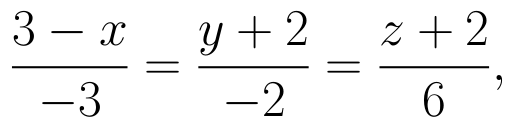then, find the direction cosines of a line parallel to AB. (View Answer Video)

Question 2 : Find the distance between the point (5, 4, -6) and its image in xy-plane. (View Answer Video)

Question 3 : Write the distance between the parallel planes 2x - y + 3z = 4 and 2x - y + 3z = 18. (View Answer Video)

Question 4 : Write the vector equation of a line passing through the point (1, -1, 2) and parallel to the line whose equations are,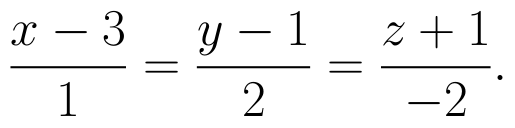(View Answer Video)

Question 5 : Find the equation of plane passing through the line of intersection of the planes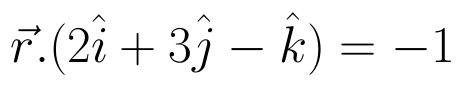and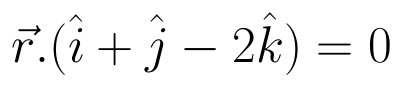and passing through the point (3, -2, -1). Also, find the angle between the two given planes. (View Answer Video)

### Differential Equations

Question 1 : Write the sum of the order and degree of the differential equation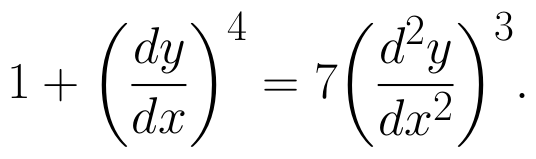(View Answer Video)

Question 2 : Show that the solution of differential equation :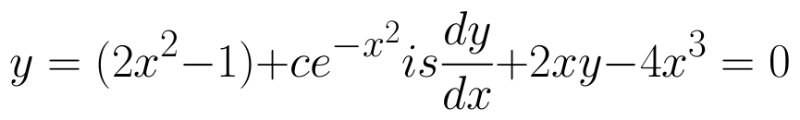(View Answer Video)

Question 3 : Solve the differential equation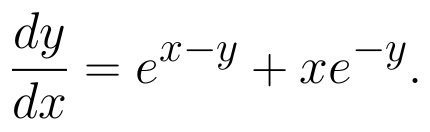(View Answer Video)

Question 4 : If m and n are the order and degree, respectively of the differential equation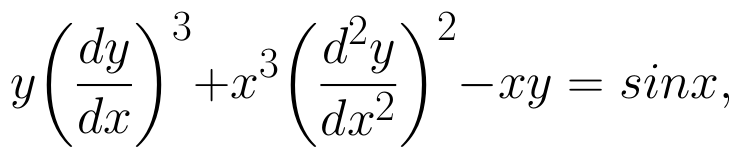then write value of m+n. (View Answer Video)

Question 5 : Solve the following differential equation :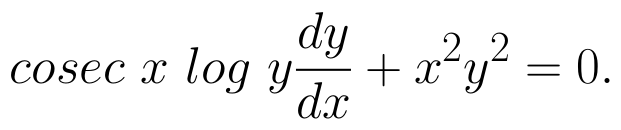(View Answer Video)

### Application of Integrals

Question 1 : Find the area of the region enclosed by the parabola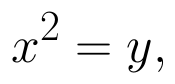the line y = x + 2. (View Answer Video)

Question 2 : Find the area of the region lying in the first quadrant and bounded by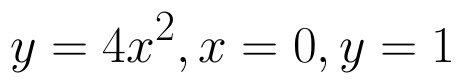and y = 4. (View Answer Video)

Question 3 : Find the area enclosed by the parabola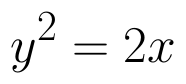and the line x - y = 4. (View Answer Video)

Question 4 : Find the area of the region bounded by the curve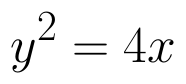and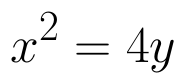. (View Answer Video)

Question 5 : Using the method of integration find the area of the region bounded by lines:
2x + y = 4, 3x - 2y = 6 and x - 3y + 5 = 0.
Question 1 : Find the unit vector in the direction of the vector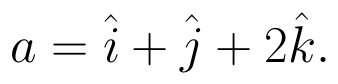(View Answer Video)
Question 3 : Find the direction of the vector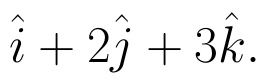(View Answer Video)
Question 4 : Find a vector in the direction of vector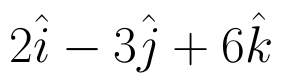which has magnitude 21 units.  (View Answer Video)
Question 5 : Find the value of x and y so that the vectors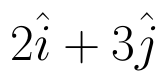and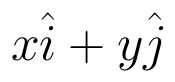are equal. (View Answer Video)